# An experiment to prove the flat earth theory conversely proves that the earth is a sphere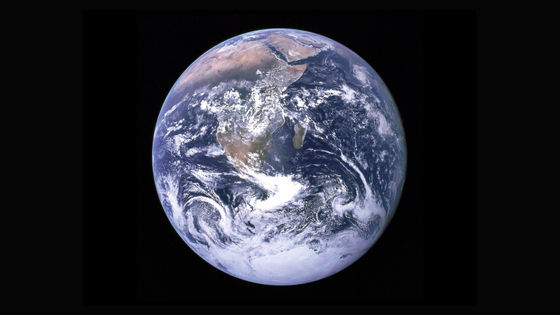Geran Campanella, who believes in the

flat earth theory, conducted an experiment to prove the flat earth theory in the movie ' Behind the Curve - Flat Earth Theory - ', but on the contrary, the earth is a sphere We obtained results that prove that

Interesting | MCToon
https://mctoon.net/interesting/

In Mr. Campanella's experiment, a camera was installed on one side of the point at 0 m above sea level, and an assistant with a bright light stood on the other side 3 miles (about 4.8 km) away. Two boards with holes were placed in between, and the height of the camera, light, and hole was 17 feet (about 5.1 m) from the ground.

If the earth is flat, the light should be reflected by the camera through the perforated plate. However, in Mr. Campanella's experiment, the light of the light was not reflected.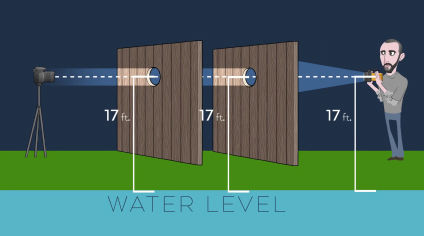Campanella then used the formula that 8 inches (about 20 cm) of light falls per square mile and predicted that 6 feet (about 1.8 m) of light would fall in 3 miles. Therefore, without changing the position of the camera and the hole in the board, the height of the light was raised to 23 feet (about 7 m) and an experiment was performed, but the light of the light was not reflected.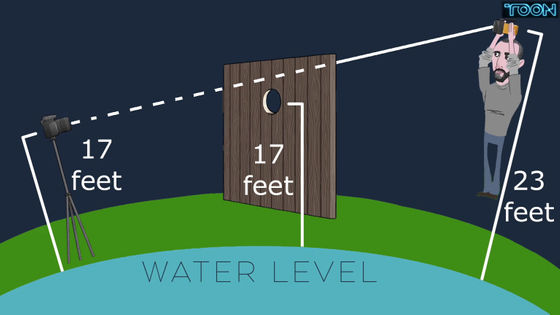According to science blog MCToon , Mr. Campanella's prediction that light would fall six feet in three miles was correct, but the hole in the board between them was too low, so it failed.

In order to actually verify the correct height of the light, it is necessary to follow the path of the light and calculate the lowest point of the path of the light over the entire 3 miles to the camera.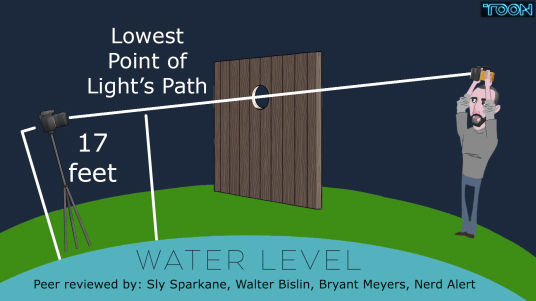MCToon creates a program that runs on Python and performs calculations. The lowest point is halfway between the camera and the board, 2.25 miles (about 3.6 km) from the light.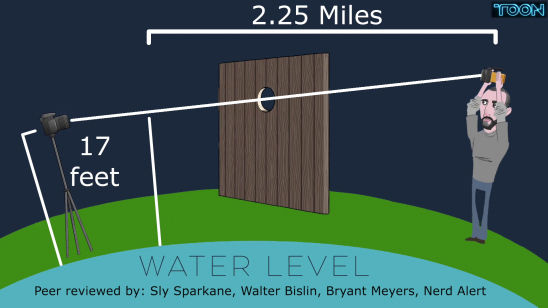Furthermore, using the formula above, the minimum height at which light falls is 40.5 inches (about 1 m).

At 0.75 miles (about 1.2 km) after the lowest point, the light path rises 4.5 inches (about 11.4 cm) to the height of the camera, so that the light reaches the camera.

However, in reality, it is affected by refraction that changes depending on the weather. The higher the refractive index , the lower the expected light height.

Assuming +0.167, which is the index of refraction of the earth at night, the light drop including refraction is 6.67 inches per square mile, and the lowest light drop height is 33.76 inches. . The rise from the lowest height to the camera is 3.75 inches (about 10 cm), and the distance from the light to the camera including refraction is calculated to be 2.5 feet (about 76 cm).

Therefore, in order to project the light to the camera at a height of 17 feet, it is necessary to install the light at a height of 19.5 feet (about 5.9 m) from the ground.

Under the flat Earth theory, the light never reached the camera in this experiment, thus proving the spherical Earth theory. However, Mr. Campanella is considering re-experimenting at a higher altitude to prove the flat earth theory, assuming that the light did not reach the camera due to weeds.

in Science, Posted by log1r_ut